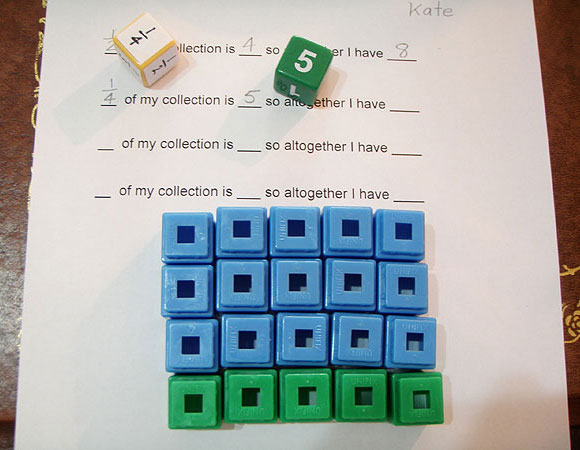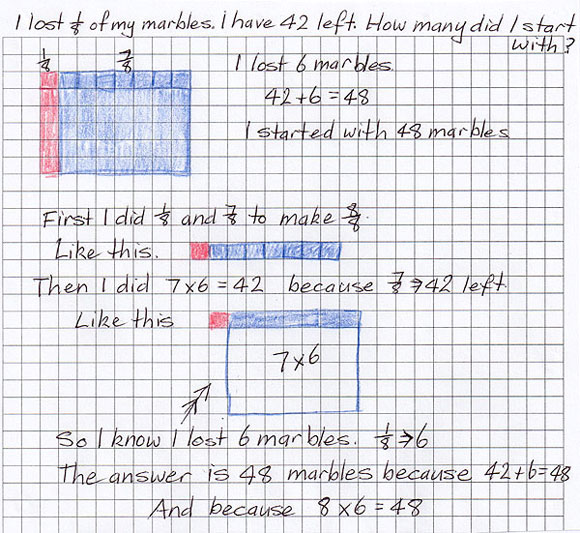# Part and wholes

In years 3 and 4, students use two dice, one labelled 1 to 6 (called the whole-number die) and the other labelled with fractions (called the fraction die). The fraction die has fractions appropriate to the year level (e.g. for year 3 the fractions could be $$\frac{1}{2}$$, $$\frac{1}{4}$$, $$\frac{1}{8}$$, $$\frac{1}{3}$$, $$\frac{1}{5}$$).

Pairs of students roll the two dice to generate numbers to insert into the sentence:

(fraction roll) of my collection is (whole-number roll) so altogether I have ____Example: One quarter of my collection is 5 so altogether I have __

Students model the problem, using counters to make a group of five.

They then make more groups of five until there are four equal groups, and calculate the total.

Teachers should:

• encourage the formation of arrays to make the relationship with factors and multiples more obvious
• discuss strategies for working out the total without having to build the array
• ask what other fractions can be determined from the array, such as
$$\frac{3}{4}$$ of 20 = 15
$$\frac{1}{5}$$ of 20 = 4

In year 6, students solve problems to determine fractions of collections and multiples of those fractions. For example:

I lost $$\frac{1}{8}$$ of my marbles. I have 42 left. How many did I start with?Student work sample

Discuss with the class:

• possible strategies for solving the problems
• the effectiveness of various strategies.

For students not confident in factors and multiples, creating arrays on grid paper can provide scaffolding for finding strategies.